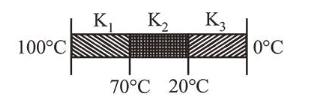# Three rods of identical cross-section and lengths are made of three different materials`
Question:

Three rods of identical cross-section and lengths are made of three different materials of thermal conductivity $\mathrm{K}_{1}, \mathrm{~K}_{2}$ and $\mathrm{K}_{3}$, respectively. They are joined together at their ends to make a long rod (see figure). One end of the long rod is maintained at $100^{\circ} \mathrm{C}$ and the other at $0^{\circ} \mathrm{C}$ (see figure). If the joints of the rod are at $70^{\circ} \mathrm{C}$ and $20^{\circ} \mathrm{C}$ in steady state and there is no loss of energy from the surface of the rod, the correct relationship between $\mathrm{K}_{1}, \mathrm{~K}_{2}$ and $\mathrm{K}_{3}$ is:1. $\mathrm{K}_{1}: \mathrm{K}_{3}=2: 3, \mathrm{~K}_{1}<\mathrm{K}_{3}=2: 5$

2. $\mathrm{K}_{1}<\mathrm{K}_{2}<\mathrm{K}_{3}$

3. $\mathrm{K}_{1}: \mathrm{K}_{2}=5: 2, \mathrm{~K}_{1}: \mathrm{K}_{3}=3: 5$

4. $\mathrm{K}_{1}>\mathrm{K}_{2}>\mathrm{K}_{3}$

Correct Option: 1

Solution:

(1) As the rods are identical, so they have same length $(l)$ and area of cross-section $(A)$. They are connected in series.

So, heat current will be same for all rods.

Heat current $=\left(\frac{\Delta Q}{\Delta t}\right)_{A B}=\left(\frac{\Delta Q}{\Delta t}\right)_{B C}=\left(\frac{\Delta Q}{\Delta t}\right)_{C D}$

$\Rightarrow \frac{(100-70) K_{1} A}{l}=\frac{(70-20) K_{2} A}{l}=\frac{(20-0) K_{3} A}{l}$

$\Rightarrow K_{1}(100-70)=K_{2}(70-20)=K_{3}(20-0)$

$\Rightarrow K_{1}(30)=K_{2}(50)=K_{3}(20)$

$\Rightarrow \frac{K_{1}}{10}=\frac{K_{2}}{6}=\frac{K_{3}}{15} \Rightarrow K_{1}: K_{2}: K_{3}=10: 6: 15$

$\Rightarrow K_{1}: K_{3}=2: 3 .$